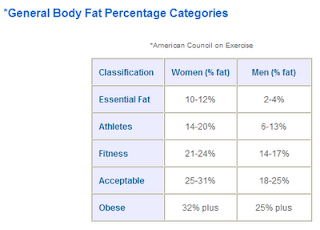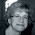## Sunday, February 15, 2009

### My New Body Fat GadgetA few weeks ago I bought the Omron Body Fat Analyzer.  It was on sale and I couldn't resist a cool gadget.

By knowing my actual body fat percentage, I have a more scientific basis to gage my progress in weight loss and also a new way to establish a goal weight.  You know me... I like my facts based in science.

Using the BMI scale is fine for determining a healthy range for a goal weight.  But it's a general guideline and does not take into account an individual person's body composition.  It's also a good way to determine if my exercise is "working" -- Is it building muscle and burning fat?So to understand where I need to be, I have to understand what a healthy body fat percent is for me.  According to the table here from the American Council on Exercise ... my goal is to be at a body fat percentage of about 24%.  This would put me in the Fitness category.

That translates to a new goal weight of 160. Which actually is only 9 pounds lower than what my "first goal weight" has been since the beginning.  Below is the math formulas you need to figure out what your body fat percentage means and how to set your goal weight based on the percentage system (instead of the BMI system).

This is a copy/paste from an email I sent to my support group girls in helping them understand how to do the math.  Figured I'd post it here too so it's at my fingertips when I need the math formulas.

---

I thought I'd pass along some formulas and calculations to understand what the Body Fat Percentage numbers mean and how you can use that information for real-world goals. For the examples below, let's use my numbers...
• My current weight: 190
• My current body fat: 36%
• My goal body fat: 24% (Fitness Category)
First, I need to know how much my lean muscle mass weighs.

Here's the basic formula:

weight - (weight x CurrentBodyFatPercentage) = lean muscle mass
• 190 X 36% = 68.4 pounds --- this is how much the fat on my body by weighs. Let's round it to 68 for ease.
• 190 - 68 = 122 pounds --- this is how much my lean muscle mass weighs (including bones, muscle, organs, skin)
Now I want to know what I would weigh if I had 24% body fat.

The secret formula is:
lean muscle mass / (1 - GoalBodyFatPercentage) = new weight goal
• 122 / (1 - .24) = 160

What does all this mean?
CurrentWeight - NewGoalWeight = PoundsLeftToLose
• 190 - 160 = 30lbs That's all I have to lose in order to hit that category of "Fitness"
HOWEVER --- as we lose weight through exercise, our lean muscle mass will change - my 122 might end up being 126 by the time I reach 24%. This means I have to do this formula everytime I test my body fat percentage again.

What's interesting is that when I use the body fat percentage to determine my ideal body weight, it contradicts what the BMI chart says is ideal. At 160, I will still be "overweight" according to BMI. And technically right in the middle of the "Normal" BMI range, I would weigh 134... which would leave me with only 9% body fat - way below the healthy essential range.

To make life easier, I created a MS Excel Spreadsheet with the formulas already programmed in for you (download below). That way you don't have to actually use your brain if you don't want to. Have fun figuring it all out.

1.2.